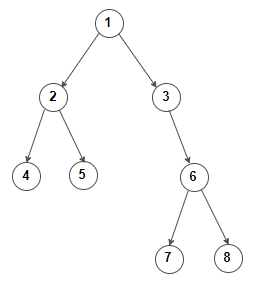# Find next node in same level for given node in a binary tree

Given a binary tree and a node in it, write an efficient algorithm to find its next node in same level as given node.

For example, consider below binary treeNext node of 2 is 3
Next node of 5 is 6
Next node of 7 is 8
Next node of 8 is null

Simple solution would be to perform level order traversal of the tree. We can modify level order traversal to maintain level number of each node. Then if the given node is found, we return its immediate right node which is present at the same level.

C++ implementation –

The time complexity of above solution is O(n) and auxiliary space used by the program is O(n).

We can also solve this problem by using constant auxiliary space and in linear time. The idea is to traverse the tree in preorder fashion and search for the given node. Once the given node is found, we mark its level number. Then the first node encountered at same level is the next right node.

C++ implementation –

The time complexity of above solution is O(n) and need O(h) extra space for the call stack where h is the height of the tree.(1 votes, average: 5.00 out of 5)Loading...

Please use our online compiler to post code in comments. To contribute, get in touch with us.
Like us? Please spread the word and help us grow. Happy coding 🙂